# Mathematics Support

### Expressing Geometric Relationships

Measurement in Two and Three Dimensions

Angle Relationships

## Measurement in Two and Three Dimensions

### Students will be able to...

• Draw (freehand, with ruler and protractor, and with technology) geometric shapes with given conditions. Focus on constructing triangles from three measures of angles or sides, noticing when the conditions determine a unique triangle, more than one triangle, or no triangle.
• Describe the two-dimensional figures that result from slicing three-dimensional figures, as in plane sections of right rectangular prisms and right rectangular pyramids.
• Know the formulas for the area and circumference of a circle and use them to solve problems; give an informal derivation of the relationship between the circumference and area of a circle.
• Solve real-world and mathematical problems involving area, volume and surface area of two- and three-dimensional objects composed of triangles, quadrilaterals, polygons, cubes, and right prisms.

The Common Core State Standards require a balance of three fundamental components that result in rigorous mathematics acquisition: deep conceptual understanding, procedural skill, and mathematical applications and modeling.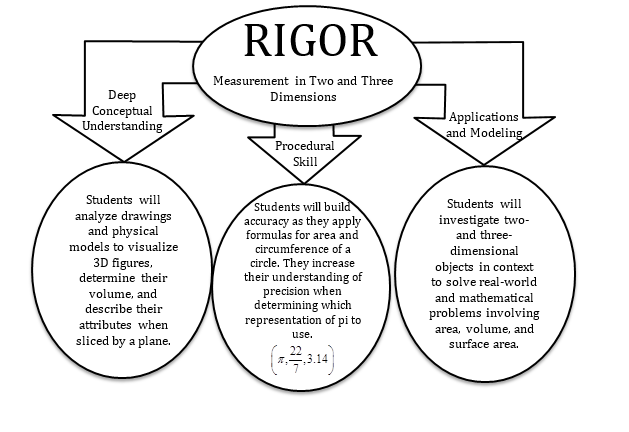### In school, your child will…

• Draw (freehand, with ruler, and with technology) geometric shapes with given conditions.

Draw a segment AB 1 cm. in length. Draw a circle whose radius is segment AB.

• Describe the two-dimensional figures that result from slicing three-dimensional figures, as in plane sections of right rectangular prisms and right rectangular pyramids.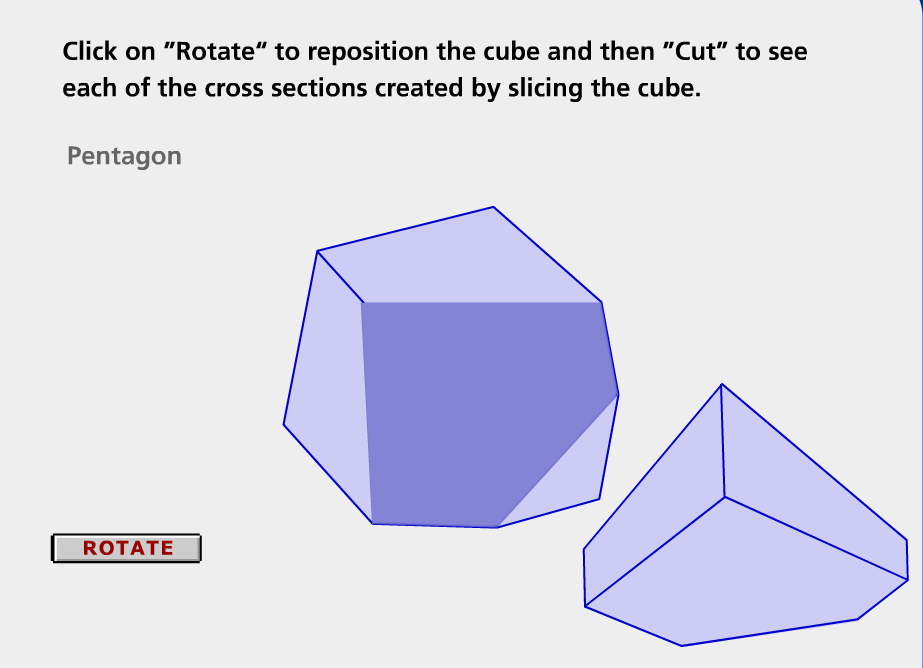• Know the formulas for the area and circumference of a circle and use them to solve problems; give an informal derivation of the relationship between the circumference and area of a circle.

Describe how the two illustrations can be used to describe the relationship between the area and circumference of a circle.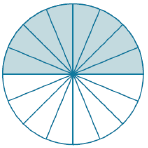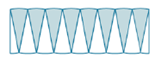• Solve real-world and mathematical problems involving area, volume and surface area of two- and three-dimensional objects composed of triangles, quadrilaterals, polygons, cubes, and right prisms.

The diagram represents the area of the placemat. Each square on the grid measures 4 inches in length. Find the area of the entire placemat.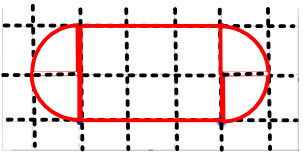### At home, your child can...

• Draw (freehand, with ruler, and with technology) geometric shapes with given conditions.

Investigate automotive brand logos that are composed of polygons and circles. Practice measuring and drawing the logos using everyday tools, such as rulers and string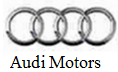• Solve real-world and mathematical problems involving surface area of two-dimensional objects composed of triangles, quadrilaterals, polygons, and circles.

For example, examine the shape of a landscaped flowerbed. Determine the shapes that compose the figure. Discuss the strategy that can be used to determine the area of the flowerbed.

*Additional Practice links support C2.0 content, but may use vocabulary or strategies not emphasized by MCPS.

## Angle Relationships

### Students will be able to...

*Instructional videos in the hyperlinks above are meant to support C2.0 content, but may use vocabulary or strategies not emphasized by MCPS.

The Common Core State Standards require a balance of three fundamental components that result in rigorous mathematics acquisition: deep conceptual understanding, procedural skill, and mathematical applications and modeling.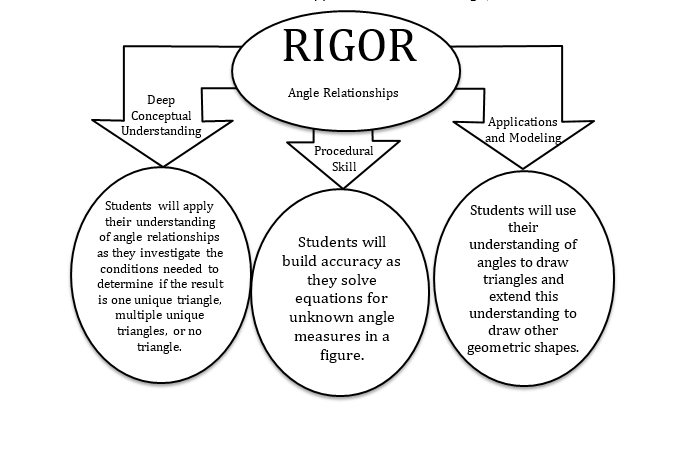### In school, your child will…

• Draw (freehand, with ruler and protractor, and with technology) geometric shapes with given conditions. Focus on constructing triangles from three measures of angles or sides, noticing when the conditions determine a unique triangle, more than one triangle, or no triangle.

Use your protractor to measure the angles of triangles A and B.  Use your ruler to measure the side lengths of triangles A and B (in cm).  Label the angle measures and side lengths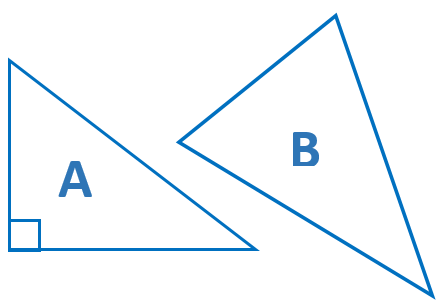• Use facts about supplementary, complementary, vertical, and adjacent angles in a multi-step problem to write and solve simple equations for an unknown angle in a figure.

Write and solve equations to determine the unknown angle measures below: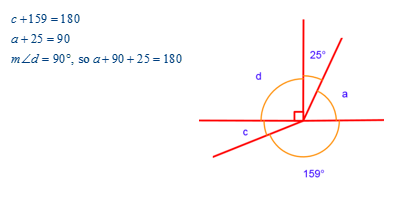### At home, your child can...

• Practice identifying and measuring sketched angles using a protractor.  Practice rotating the interactive protractor into proper position to measure each given angle at http://www.mathplayground.com/measuringangles.html.
• Investigate a local street system design using a street map or by visiting Google© maps.

• Locate pairs of intersecting roads and determine the angle relationship that exists. Use a protractor to confirm the relationship.
• Determine if any series of intersecting roads results in a triangle and identify the type of triangle that is represented.
• Locate and label the different angles on the map and use a protractor for measuring.

• Examine a soccer field and see how angle measures are at work. Visit the CK12 PLIX (Play Learn Interact Xplore): Corner Kick. To access the PLIX, you will need to create a free user account.